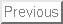This exam consists of 2 parts. Part I contains 10 short ``grab-bag'' questions, each worth 4 points. Part II contains 5 longer problems, each worth 12 points. This test is CLOSED BOOK. However, each student is allowed to bring two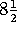by 11 sheets of prepared notes into the test. Other than these sheets, you are only allowed your pencils and pens and a NON-GRAPHICS calculator.

Evaluate the following derivatives, integrals, and limits, and simplify the following expressions involving exponentials and logs.

For problems A-D, you do NOT have to reduce your answers to the simplest possible forms. Any correct answers will do.

However, for problems E-J, I do want answers in the simplest possible form. For example, if the answer is zero, then writing ``ln(1)'' (which DOES equal zero, of course) will only get you partial credit. Also, you should show your reasoning. Just pulling numbers from a calculator will get you NO credit.

Some of the limits may not exist. If they don't exist, write ``DNE'' or ``does not exist'' in the box.

A. Find F(x), where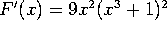and F(0)=5.

B. Evaluate: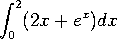.

C. Find f'(x), where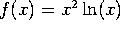D. Find f'(x), where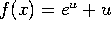and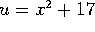.

E. Find f'(x), where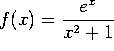F. Evaluate (and simplify):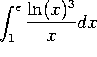G. Evaluate (and simplify):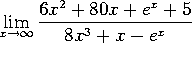H. Evaluate (and simplify):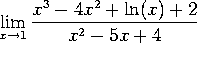I. Simplify: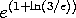J. Simplify: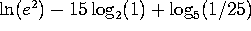.

This part consists of 5 longer problems, each worth 12 points.

Problem 1. A certain professor of mathematics overeats during a 12-day holiday period and gains weight. The rate at which he gains weight is given by the formula: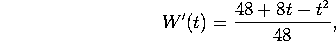where his weight, W(t), is measured in pounds, and the time t is measured in days. (That is, the holiday starts at t=0 and ends at t=12).

Note that parts (a) and (b) below can be done independently of each other. Part (a) involves differentiation, while part (b) involves integration.

a) At what time is the professor gaining weight the fasting? How fast is he gaining at that time?

b) If he starts the holidays weighing 160 pounds, what is his weight at the end of the holidays?

Problem 2. Consider the function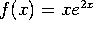.

a) Find all the partition points of f. For what values of x is f(x) positive? Find all the horizontal asymptotes (if any) and all the vertical asymptotes (if any).

b) Compute f'(x). Find all the critical points, and for each one say whether it is a local minimum, a local maximum, or neither. For what values of x is f increasing?

c) Compute f''(x). Find all the points of inflection. For what values of x is the graph of f concave up?

d) Sketch the graph of f(x).

Problem 3. Suppose that a colony of bacteria is growing in your lungs (cough, cough). The number of bacteria, N(t), is growing according to the formula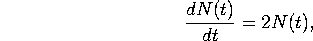where t is time, measured in hours. At midnight (t=0) there are 10 bacteria in your lungs (i.e. N(0)=10).

a) How many bacteria will there be at time t? (This answer should be a formula)

b) How many will there be at 8 AM? (This answer should be a number)

c) At what time will there be 1000 bacteria?

4. a) Consider the curve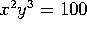. Find dy/dx at the point (10,1).

b) The pressure and volume of gas in a piston are related by the formula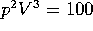, where p denotes pressure and V denotes volume. When p=10 and V=1, the volume is increasing at a rate of dV/dt=2. At what rate is the pressure changing?

5. Let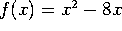. Let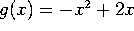.

a) Where do the curves y=f(x) and y=g(x) meet?

b) Find the area between the two curves.